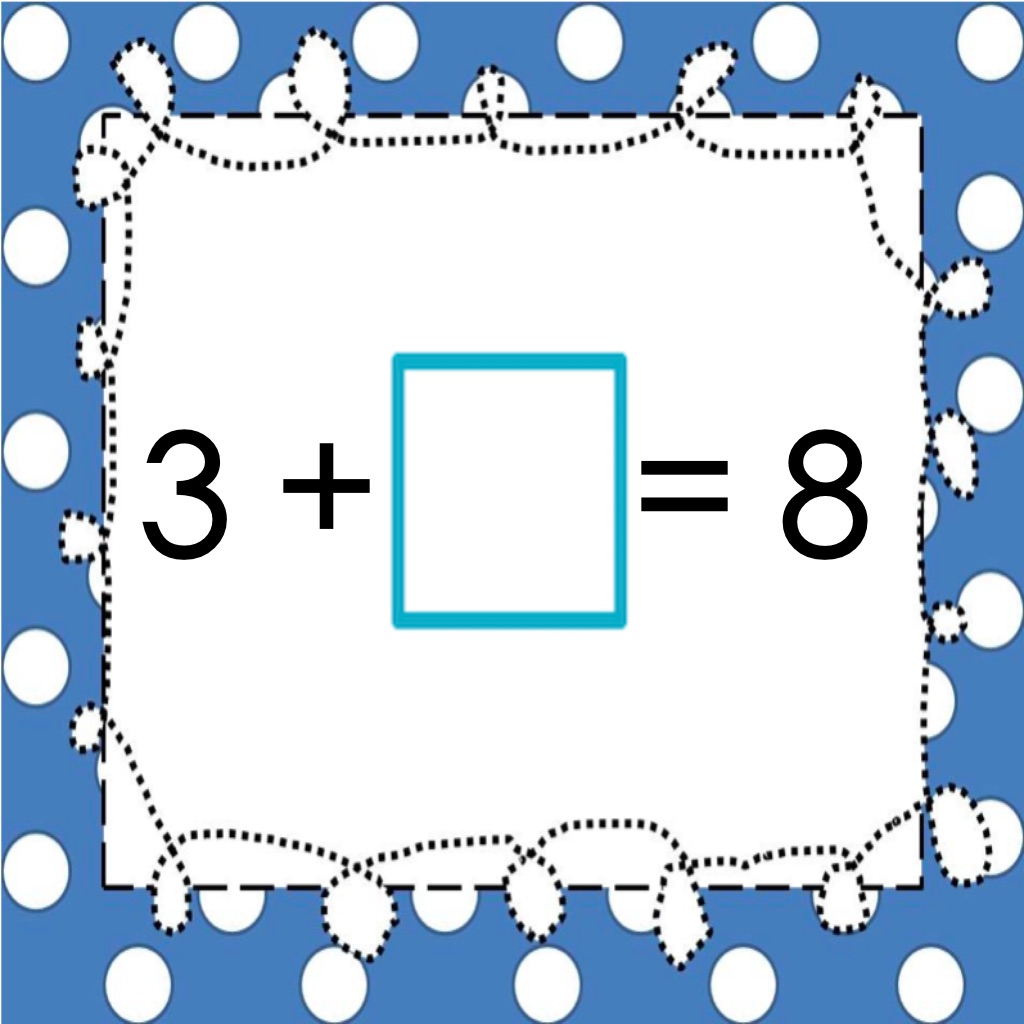Addition and Subtraction ~ Complete the Equation Free# Addition and Subtraction ~ Complete the Equation Free

## Anne Gardner

2.0 for iPhone, iPad
Free
0

0 Ratings

Release Date

2015-02-26

Size

6.2 MB

### Screenshots for iPhone

iPhone
More Screenshots for iPad
Addition and Subtraction ~ Complete the Equation Free Description
This app is designed to help students master the following Common Core Math Standards:

CCSS.MATH.CONTENT.1.OA.B.3 - Apply properties of operations as strategies to add and subtract.2 Examples: If 8 + 3 = 11 is known, then 3 + 8 = 11 is also known. (Commutative property of addition.) To add 2 + 6 + 4, the second two numbers can be added to make a ten, so 2 + 6 + 4 = 2 + 10 = 12. (Associative property of addition.)

CCSS.MATH.CONTENT.1.OA.D.8 - Determine the unknown whole number in an addition or subtraction equation relating three whole numbers. For example, determine the unknown number that makes the equation true in each of the equations 8 + ? = 11, 5 = _ - 3, 6 + 6 = _.

CCSS.MATH.CONTENT.1.OA.C.6 - Add and subtract within 20, demonstrating fluency for addition and subtraction within 10.

First grade students need scaffolded experiences to develop problem these solving skills. This app includes 240 equation cards that are presented in 6 levels of increasing complexity.

First grade students need scaffolded experiences to develop problem solving skills. The full app includes 240 equation cards that are presented in 6 levels of increasing complexity- this version has just level 1.

This app is also excellent for helping second and third grade students develop problem solving skills and fluency with addition and subtraction facts.

The levels are:

Subtraction within 10
Mixed +/- within 10
Subtraction within 20
Mixed +/- within 20

We hope your students enjoy these games. If you have questions or feedback, we’d love to hear from you. We can be reached at gardnerdevelopment@gmail.com.

***Also available***
-Comparing Numbers ~ Greater Than, Less Than, or Equal

-Comparing Decimals ~ Greater Than, Less Than, or Equal

-True or False Math Equations

-Reading Comprehension Passages with Multiple Choice Questions ~ Guided Reading Level C

-Reading Comprehension Passages with Multiple Choice Questions ~ Guided Reading Level D

-Spanish Reading Comprehension Level 3
Addition and Subtraction ~ Complete the Equation Free 2.0 Update
2015-02-26 Version History
iPhone 6/6 Plus support!
More
Price:
Free
Version:
2.0
Size:
6.2 MB
Genre:
Education Games
Release Date:
2015-02-26
Developer:
Anne Gardner
Language:
English
More
You May Also Like
Developer Apps